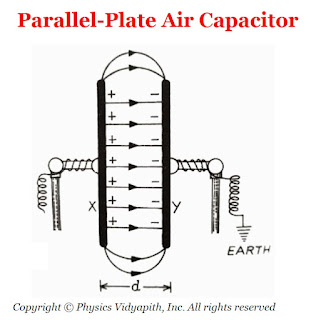### Parallel Plate Air Capacitor and Its Capacitance

Parallel Plate Air Capacitor→

A parallel-plate capacitor consists of two long, plane, metallic plates mounted on two insulating stands and placed at a small distance apart in a vacuum (or air). The plates are exactly parallel to each other.Parallel Plate Air Capacitor
Derivation of the capacitance of parallel plate capacitor in the air→

Let us consider, Two plates $X$ and $Y$ are separated at a small distance $d$ in the vacuum (or air). If the area of plates is $A$ and the plates $X$ and $Y$ have charge $+q$ and $-q$ respectively. If the surface charge density on each plate is $\sigma$ then electric field intensity at a point between two parallel plates is

$E=\frac{\sigma}{\epsilon_{\circ}}$

$E=\frac{q}{\epsilon_{\circ}A}\qquad(1) \qquad (\because \sigma=\frac{q}{A})$

The potential difference between the parallel plates capacitor in air (or vacuum) is

$V=E.d$

Now substitute the value of $E$ from equation$(1)$ in the above equation

$V=\frac{q}{\epsilon_{\circ}A}.d \qquad(2)$

The capacitance of the parallel plate capacitor in air (or vacuum) is

$C_{\circ}=\frac{q}{V} \qquad(3)$

From equation $(2)$ and equation $(3)$

$C_{\circ}=\frac{q}{\frac{q}{\epsilon_{\circ}A}.d}$

 $C_{\circ}=\frac{\epsilon_{\circ}A}{d}$

If the space between the plates is filled with some dielectric medium of dielectric constant $K$, then the electric field between the plates is

$E=\frac{\sigma}{K \: \epsilon_{\circ}}$

The Potential difference between the parallel plates when dielectric medium $(K)$ is present between them

$V=\frac{q}{K \: \epsilon_{\circ} \: A}.d$

Now, The capacitance of the parallel-plate capacitor when the dielectric medium $(K)$ is present between them

 $C=\frac{K\: \epsilon_{\circ} \: A}{d}$